# Pandas Boxplots: Everything You Need to Know to Visualize DataUpdated:

Published:

Data visualizations are a powerful tool to better understand the attributes of a data set.pandas is a Python library built to streamline processes around capturing and manipulating relational data that has built-in methods for plotting and visualizing the values captured in its data structures.

One popular method for visualizing numerical data in pandas is the boxplot. In this post, you'll learn the basics of a boxplot and see examples of boxplots in pandas.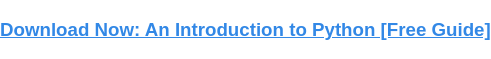## Boxplot in Pandas

A boxplot is created by calculating the quartiles of a data set, which divide the number range into four pieces based on their distribution.

To understand quartiles better, let's break each one down:

• Median: the value in the middle of the distribution
• Lower quartile: the midpoint between the median and lowest value in the range
• Upper quartile: the midpoint between the median and highest value in the range
• Lower boundary: the lowest value in the distribution
• Higher boundary: the highest value in the distribution

You can see each of these values marked on the boxplot diagram below.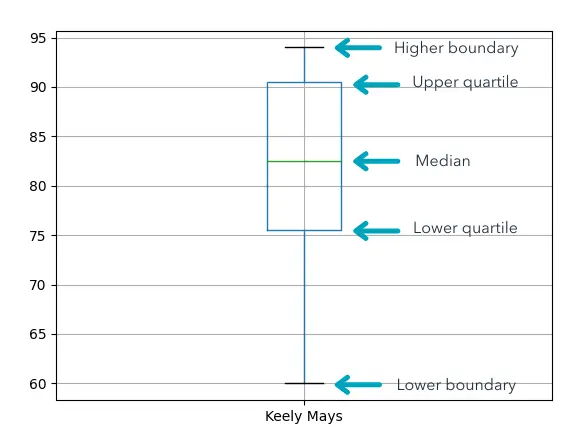Boxplots help you better understand the range of values in your data set and identify any outliers in a format that's easier to understand than the raw data.

Now that you understand what a boxplot is, let's dive into how to create one in pandas.

## Pandas Boxplot Examples

To start, you will need to import the pandas and matplotlib libraries. Specifically, you will be importing the submodule pyplot since that is where you will access the method to display your boxplot rather than importing the entire matplotlib library. The import statements for both libraries are below.

``` ```
import pandas as pd
import matplotlib.pyplot as plt
``````

Now that you have the proper tools, you can start to build your boxplots.

For these tutorials, the visualizations will be created from a DataFrame of students with columns of randomly generated grades. The DataFrame is printed to the terminal below.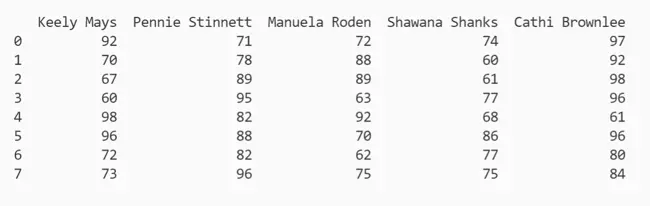Below are examples of boxplots generated from one column and multiple columns of data.

### Pandas Boxplot Single Column

For the first example, let's examine how to create a boxplot for a single column of data, also known as a Series. In this code, you are generating a boxplot from the column named "Keely Mays":

``` ```
stud_bplt = stud_df.boxplot(column = 'Keely Mays')
stud_bplt.plot()
plt.show()
``````

To start, you call the .boxplot() method of the pandas library on the stud_df DataFrame. You assign the newly calculated values of the boxplot to the stud_bplt variable.

Next, you call .plot() to plot the boxplot values in stud_bplt into a chart in the pyplot interface. Then, you call the .show() method on the pyplot module to show the interface with the now plotted boxplot.

The output is below.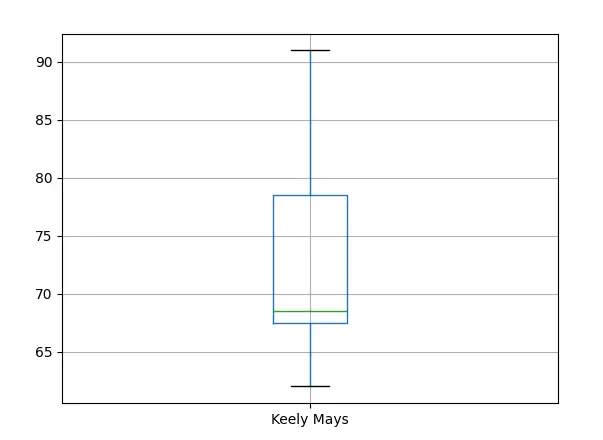The distribution of the column labeled "Keely Mays" is now captured in a boxplot. You can see the boundaries, upper and lower quartiles, and median of their grades.

Now that you understand the basic workflow, let's look at how to compare multiple columns side by side.

### Pandas Boxplot Multiple Columns

Boxplots are not limited to portraying single columns; a major use case for boxplots is to compare related distributions. You can easily expand the scope of your boxplot to multiple columns of data by providing a list of column names to the .boxplot method:

``` ```
stud_bplt = stud_df.boxplot(column = [
'Keely Mays',
'Pennie Stinnett',
'Manuela Roden',
'Shawana Shanks',
'Cathi Brownlee'
])
stud_bplt.plot()
plt.show()
``````

Here, you are passing in a list of labels for the column argument. The rest of the workflow remains the same.

The output is below.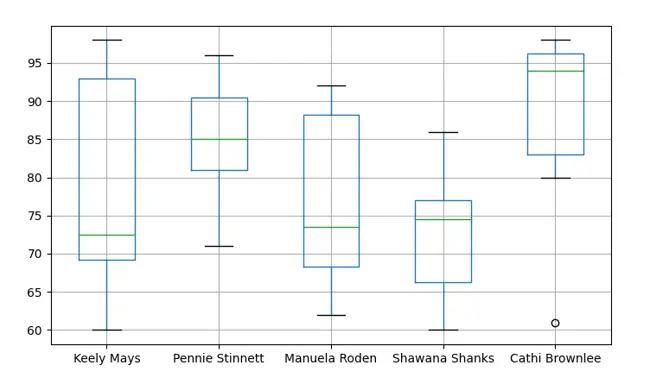Now you can see the grade distributions for all the students in the data set and how each students' grades compare with their peers. You can also note the presence of an outlier in the "Cathi Brownlee" distribution, as denoted by the bubble outside the distribution.

The column argument is not the only parameter available under the .boxplot method. Let's examine some customizations next.

## Pandas Boxplot Customizations

The pandas library provides multiple arguments for you to further customize the display of your boxplot. Let's review a few of the more popular options.

### 1. Pandas Boxplot Color

You might decide that the default coloring of the boxplot distribution could be improved. To override it, you can use the color argument to modify the visualization's appearance:

``` ```
stud_df.boxplot(column = 'Keely Mays', color = 'red')
``````

Here, you declare the color argument and set it equal to "red" when calling .boxplot() in pandas.

The output is below.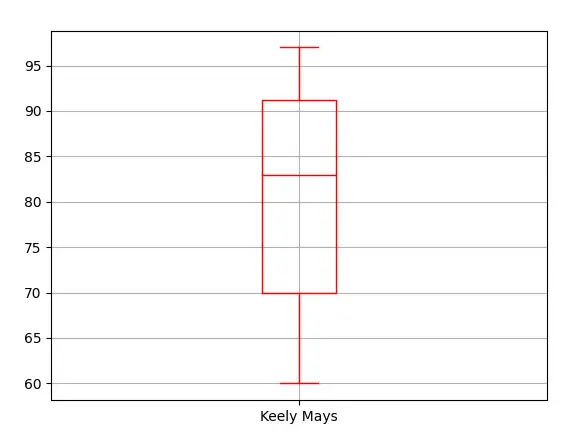Your boxplot is now a distinctive red. You can set the color to any other basic named color. Think ROY G BIV: red, orange, yellow, green, blue, indigo, violet.

The next customization allows you to add a title to your chart.

### 2. Pandas Boxplot Title

Titles help users quickly understand what they are looking at. You can add a title to your boxplot by turning to the pyplot module.

In this code, you are creating a boxplot as normal, but this time you add an extra step:

``` ```
stud_bplt = stud_df.boxplot(column = 'Keely Mays')
stud_bplt.plot()
plt.show()
``````

Here, you call the .title() method to assign the boxplot the title "Keely Mays Grade Distribution." Then you call .show() as usual to see the final result: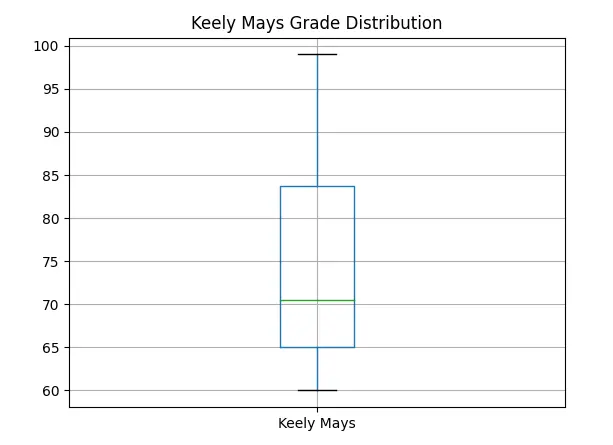Now you have a title to inform any newcomers what this boxplot represents so they can understand it quicker.

This final customization returns to the arguments of the .boxplot method.

### 3. Pandas Boxplot Label Font Size

You may want to modify the default font size of the boxplot labels. This can make the boxplot more accessible and easier to read.

``` ```
stud_bplt = stud_df.boxplot(column = 'Keely Mays', fontsize = 15)
stud_bplt.plot()
plt.show()
``````

Here, you are increasing the font size of the boxplot labels to 15. This value correlates closely to point size, as you can see below: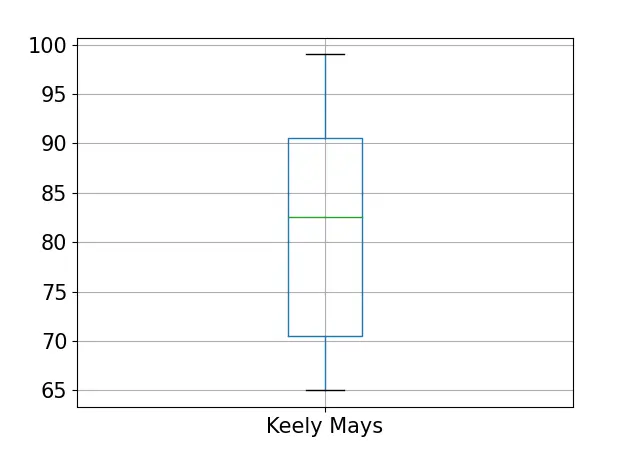Now both the column label and grid labels are easier to read.

Boxplots are a powerful visualization tool to dive deeper into numerical data. They reveal the distribution of values in ranges and any outliers within your data sets. By leveraging the full capabilities of the pandas and matplotlib libraries, you can customize your boxplots to meet your business's needs and improve decision making.Topics: What Is Python?

## Related Articles

We're committed to your privacy. HubSpot uses the information you provide to us to contact you about our relevant content, products, and services. You may unsubscribe from these communications at any time. For more information, check out our Privacy Policy.

A guide for marketers, developers, and data analysts.